Composite of consecutive maps of cellular chain complex is zero

Statement

Statement independent of the language of topological spaces

Suppose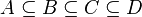$A \subseteq B \subseteq C \subseteq D$ are topological spaces, each having the subspace topology from the next. Suppose$n$ is a (nonnegative, though it doesn't matter) integer. Consider the following three cases of the long exact sequence of homology of a pair (we write different parts of the long exact sequences because we need different parts for the later steps):

• The pair$(D,C)$: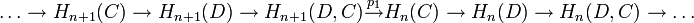$\ldots \to H_{n+1}(C) \to H_{n+1}(D) \to H_{n+1}(D,C) \stackrel{p_1}{\to} H_n(C) \to H_n(D) \to H_n(D,C) \to \ldots$

• The pair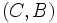$(C,B)$:$\ldots \to H_{n+1}(C,B) \to H_n(B) \to H_n(C) \stackrel{p_2}{\to} H_n(C,B) \stackrel{p_3}{\to} H_{n-1}(B) \to H_{n-1}(C) \to H_{n-1}(C,B) \to H_{n-2}(B) \to \ldots$

• The pair$(B,A)$:$\ldots \to H_n(B,A) \to H_{n-1}(A) \to H_{n-1}(B) \stackrel{p_4}{\to} H_{n-1}(A,B) \to H_{n-2}(A) \to \ldots$

Define:

•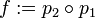$f := p_2 \circ p_1$
•$g := p_4 \circ p_3$

Then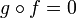$g \circ f = 0$.

Statement in terms of cellular homology

This is a special case of the previous statement, where$A = X^{n-2}, B = X^{n-1}, C = X^n, D = X^{n+1}$ are the successive skeleta of a cellular filtration of a topological space$X$. In this case, the map$f$ defined above is the boundary map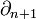$\partial_{n+1}$ and the map$g$ defined above is the boundary map$\partial_n$ in the Cellular chain complex (?). The statement is thus that$\partial_{n+1} \circ \partial_n = 0$, i.e., the cellular chain complex is indeed a chain complex.

Proof

The proof is a one-liner: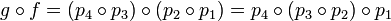$g \circ f = (p_4 \circ p_3) \circ (p_2 \circ p_1) = p_4 \circ (p_3 \circ p_2) \circ p_1$. The intermediate composite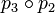$p_3 \circ p_2$ is zero since it is a composite of successive maps in a long exact sequence of homology. Hence, the overall composite is the zero map.